Sequential Simplex Optimization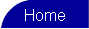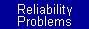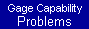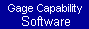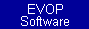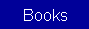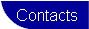After experimentation has been completed, and a process or design has been optimized, it is not uncommon for the experimentation team to consider the problem to be solved. In many cases, the optimum solution does not stay optimum; equipment changes as it ages, the composition of components change over time, and many other factors make small changes that affect the response variable. Evolutionary Operation (EVOP) is a method of addressing the constant small changes in a process in attempt to maintain an optimum level. Experimentation is carried out on a near continuous basis by introducing small changes to the system.

One of the most popular methods of EVOP is sequential simplex optimization. The simplex is a geometric figure with the number of vertexes equal to the number of factors plus one. A simplex with one factor is a line, a simplex with two factors is a triangle, etc. The simplex is a simplistic model of the surface. a new simplex is formed by eliminating the vertex with the worst response and replacing it by projecting it through the average coordinates of the remaining vertexes. A new experiment is performed with the factor levels determined by the coordinates of the new vertex, and the process is repeated until an optimum response is found. Using the sequential simplex method optimization has two advantages over using factorial designed experiments for EVOP.

1. The number of trials in the initial simplex is k+1 (k is the number of experimental factors), while a factorial approach has at least 2k and possibly 3k or 4k.
2. Only one new trial is required to move to a new area in the space defined by the factors; while a factorial design requires at least 2k-1 trials. An example of a sequential simplex optimization is shown in the figure below.To compute the coordinates of the new vertex (R), refer to the following table, where B is the vertex with the best response, N is the vertex with the next best response, and W is the vertex with the worst response. This table shows an example for a 3 factor optimization (k = 3). The logic is the same for any number of factors.

 A B C Result Next Best 20 20 20 425 Best 20 30 20 503 Other 30 20 20 378 Sum 70 70 60 Sum/k 23.3 23.3 20 Worst 20 20 15 215 Sum/k-W 3.3 3.3 5 R 26.7 26.7 25 (Sum/k-W)/2 1.7 1.7 2.5 Cw 21.7 21.7 17.5 Cr 25 25 22.5 E 30 30 30

The optimum point is never reached in the figure above because the size of the individual simplex is too large. If the simplex size is too small, an excessive number of steps is required to reach the optimum point. This problem has been solved by using a variable size simplex. Instead of a simple reflection (R), there are other options:

• double the length of the reflection (E),
• reduce the length of the expansion by 50% (Cr),
• produce the reflection in the opposite direction (W), and
• produce the reflection in the opposite direction but at 50% of the normal length (Cw).

The additional rules for determining the location of the new vertex are:

• If the response for the new vertex (R) is better than the response for the next best vertex (N), but worse than the response of the best (B) vertex, then use R as the new vertex.
• If the response for the new vertex (R) is better than the response of the best vertex (B), then compute E = P + 2(P-W).
• If the response of E is better than the response of B, use E as the new vertex; if not, use R as the new vertex.
• If the response for the new vertex (R) is worse than the response of the next best vertex (N) but better than the response of the worst vertex (W), then compute Cr = P + 0.5(P-W) as the new vertex.
• If the response for the new vertex (R) is worse than the response of the worst vertex (W), then compute Cw = P - 0.5(P-W) as the new vertex.

Example

Consider the parameter Y determined from the function Starting with the vertices

Y =  40A + 35B - 15A2 - 15B2 + 25AB

Solution

Starting with the vertices

 Vertex A B Response 1 100 100 -42,500 2 100 120 -57,800 3 120 120 -63,000

The response Y is maximized using a variable-step sequential simplex as shown below.

 Start Coordinates A B Response Rank Best (B) 100 100 -42500 B Next Best (N) 100 120 -57800 N Sum 200 220 P bar 100 110 Worst (W) 120 120 -63000 W P bar - W -20 -10 Next Vertex (R) 80 100 -39300 R Compute E E = 60 90 -34950 Use E Step 2 Coordinates A B Response Rank Best (B) 60 90 -34950 B Next Best (N) 100 100 -42500 N Sum 160 190 P bar 80 95 Worst (W) 100 120 -57800 W P bar - W -20 -25 Next Vertex (R) 60 70 -17650 R Compute E E = 40 45 -6200 Use E Step 3 Coordinates A B Response Rank Best (B) 40 45 -6200 B Next Best (N) 60 90 -34950 N Sum 100 135 P bar 50 67.5 Worst (W) 100 100 -42500 W P bar - W -50 -32.5 Next Vertex (R) 0 35 -17150 R Use R Step 4 Coordinates A B Response Rank Best (B) 40 45 -6200 B Next Best (N) 0 35 -17150 N Sum 40 80 P bar 20 40 Worst (W) 60 90 -34950 W P bar - W -40 -50 Next Vertex (R) -20 -10 -3650 R Compute E E = -60 -60 -22500 Use R Step 5 Coordinates A B Response Rank Best (B) -20 -10 -3650 B Next Best (N) 40 45 -6200 N Sum 20 35 P bar 10 17.5 Worst (W) 0 35 -17150 W P bar - W 10 -17.5 Next Vertex (R) 20 0 -5200 R Use R Step 6 Coordinates A B Response Rank Best (B) -20 -10 -3650 B Next Best (N) 20 0 -5200 N Sum 0 -10 P bar 0 -5 Worst (W) 40 45 -6200 W P bar - W -40 -50 Next Vertex (R) -40 -55 -17900 R Compute Cw Cw = 20 20 -500 Use Cw Step 7 Coordinates A B Response Rank Best (B) 20 20 -500 B Next Best (N) -20 -10 -3650 N Sum 0 10 P bar 0 5 Worst (W) 20 0 -5200 W P bar - W -20 5 Next Vertex (R) -20 10 -12950 R Compute Cw Cw = 10 2.5 -481 Use Cw Step 8 Coordinates A B Response Rank Best (B) 10 2.5 -481 B Next Best (N) 20 20 -500 N Sum 30 22.5 P bar 15 11.25 Worst (W) -20 -10 -3650 W P bar - W 35 21.25 Next Vertex (R) 50 32.5 -9581 R Compute Cw Cw = -2.5 0.625 -217 Use Cw Step 9 Coordinates A B Response Rank Best (B) -2.5 0.625 -217 B Next Best (N) 10 2.5 -481 N Sum 7.5 3.125 P bar 3.75 1.5625 Worst (W) 20 20 -500 W P bar - W -16.25 -18.4375 Next Vertex (R) -12.5 -16.875 -2432 R Compute Cw Cw = 11.875 10.78125 194 Use Cw Step 10 Coordinates A B Response Rank Best (B) 11.875 10.78125 194 B Next Best (N) -2.5 0.625 -217 N Sum 9.375 11.40625 P bar 4.6875 5.703125 Worst (W) 10 2.5 -481 W P bar - W -5.3125 3.203125 Next Vertex (R) -0.625 8.90625 -1048 R Compute Cw Cw = 7.34375 4.101563 129 Use Cw Step 11 Coordinates A B Response Rank Best (B) 11.875 10.78125 194 B Next Best (N) 7.34375 4.101563 129 N Sum 19.21875 14.88281 P bar 9.609375 7.441407 Worst (W) -2.5 0.625 -217 W P bar - W 12.10938 6.816407 Next Vertex (R) 21.71875 14.25781 -1016 R Compute Cw Cw = 3.554688 4.033203 208 Use Cw Step 12 Coordinates A B Response Rank Best (B) 3.554688 4.033203 208 B Next Best (N) 11.875 10.78125 194 N Sum 15.42969 14.81445 P bar 7.714844 7.407227 Worst (W) 7.34375 4.101563 129 W P bar - W 0.371094 3.305664 Next Vertex (R) 8.085938 10.71289 162 R Compute Cr Cr = 7.900391 9.060058 255 Use Cr Step 13 Coordinates A B Response Rank Best (B) 7.900391 9.060058 255 B Next Best (N) 3.554688 4.033203 208 N Sum 11.45508 13.09326 P bar 5.727539 6.546631 Worst (W) 11.875 10.78125 194 W P bar - W -6.14746 -4.23462 Next Vertex (R) -0.41992 2.312012 -43 R Compute Cw Cw = 8.80127 8.66394 274 Use Cw Step 14 Coordinates A B Response Rank Best (B) 8.80127 8.66394 274 B Next Best (N) 7.900391 9.060058 255 N Sum 16.70166 17.724 P bar 8.35083 8.861999 Worst (W) 3.554688 4.033203 208 W P bar - W 4.796143 4.828796 Next Vertex (R) 13.14697 13.6908 101 R Compute Cw Cw = 5.952759 6.447601 268 Use Cw Step 15 Coordinates A B Response Rank Best (B) 8.80127 8.66394 274 B Next Best (N) 5.952759 6.447601 268 N Sum 14.75403 15.11154 P bar 7.377014 7.555771 Worst (W) 7.900391 9.060058 255 W P bar - W -0.52338 -1.50429 Next Vertex (R) 6.853638 6.051483 269 R Use R R = 6.853638 6.051483 269 Use R Step 16 Coordinates A B Response Rank Best (B) 8.80127 8.66394 274 B Next Best (N) 6.853638 6.051483 269 N Sum 15.65491 14.71542 P bar 7.827454 7.357712 Worst (W) 5.952759 6.447601 268 W P bar - W 1.874695 0.910111 Next Vertex (R) 9.702148 8.267822 246 R Compute Cw Cw = 6.890106 6.902657 279 Use Cw

After 16 step the best response is 279. The optimum value is 281 when A = 7.54 and 7.46.

 Engineered Software, Inc. 734-502-8582 Information@EngineeredSoftware.com